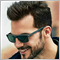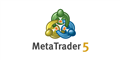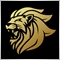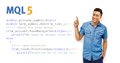Need help for Dynamic Trail Stoploss96

Hi guys. I am trying to make a dynamic trailing stop. Instead of moving the stoploss every point in profit, it only moves the stop loss after X amount in profit. And amount of stoploss must be equal to distance between Takeprofit and bid. For example When price reached WhenToTrail variable, EA must trail stoploss to the amount of TakeProfit - Bid for buy order. After that EA check TrailingStep and when price goes up x pips(step) amount of Stoploss must be equal to distance of Takprofit and Bid.

Below is my code. It is worked for external variable and I use  EditBox and got Error for static variable. Is it possible to code it differently  without static?

void DynamicTrail()
{
for(int i= OrdersTotal()-1; i>=0; i--) {
if(OrderMagicNumber()==magic)
if(Bid-OrderOpenPrice() >= WhenToTrail*pips)
{
static bool flagB = false;
if(flagB==false && OrderStopLoss() < OrderOpenPrice())
{
static double SL = 0;
double distance = (OrderTakeProfit()-OrderOpenPrice()) - (WhenToTrail*pips);  //100-30=70
double result = NormalizeDouble(distance,_Digits) - (WhenToTrail*pips);  // 70-30=40
result = NormalizeDouble(result,_Digits);

if(result==0)
SL = OrderOpenPrice()-result*pips;
else if (result>0){ //
double step = (OrderTakeProfit()-OrderOpenPrice())-(WhenToTrail*pips)-(WhenToTrail*pips); //100-60
SL = OrderOpenPrice()-NormalizeDouble(step,_Digits);
}
else if (result<0){
double step = (OrderTakeProfit()-OrderOpenPrice())-(WhenToTrail*pips)-(WhenToTrail*pips);
SL = OrderOpenPrice()+((-1)*NormalizeDouble(step,_Digits));
}

if(OrderStopLoss()<=SL) {
if(OrderModify(OrderTicket(),OrderOpenPrice(),SL,OrderTakeProfit(),0,clrNONE))
flagB = true;
else{
int err = GetLastError();
Print("Encountered an error during modification!"+(string)err+" "+ErrorDescription(err)  );
}
}

} //---When trail activated and SL Modified here at the first time

if(flagB == true)
{
static int step = WhenToTrail + PipsToUpdate;
step = step;
Print("step 0 :",step);
if((Bid-OrderOpenPrice()) >= step*pips) {

if(!OrderModify(OrderTicket(),OrderOpenPrice(),OrderStopLoss()+(2*PipsToUpdate)*pips,OrderTakeProfit(),0,clrNONE)) {
int err = GetLastError();
Print("Encountered an error during modification!"+(string)err+" "+ErrorDescription(err)  );
}
else {
step = step + PipsToUpdate;
Print("step :",step);
}

}
}

} //when trail activated and need to check steps of pipsToUpdate

}
}// end of for loop
}

And I got this idea from this video.Basic Principles - Trading Operations - MetaTrader 5
is an instruction given to a broker to buy or sell a financial instrument. There are two main types of orders: Market and Pending. In addition, there are special Take Profit and Stop Loss levels. is the commercial exchange (buying or selling) of a financial security. Buying is executed at the demand price (Ask), and Sell is performed at the...Moderator
12476

if(OrderProfit()>some_value)
{
Trailing();
}96

Marco vd Heijden:

OrderProfit() return the net profit value (without swaps or commissions) for the selected order.for example for different Symbol and lotsize it is not the same.

I need to check this:

if(Bid - OpenOrderPrice() > WhenToTrail*pips)  //first step
{
// Trail SL up equal to OrderTakeProfit()-WhenToTrail*pips

// from then on
if Bid goes PipsToUpdate= 10 pips up, check SL and then move it up to OrderTakeProfit() - Bid
}

Please watch video for sure. thanksModerator
12476

Martin Moreno:

it only moves the stop loss after X amount in profit.

This sounds like OrderProfit() to me.

Please open a job here: https://www.mql5.com/en/jobTrading applications for MetaTrader 5 to order
• www.mql5.com
The EA will use 3 EMA with different periods to identify trend direction. Also, we would need an indicator for defining price extremums, such as ZigZag (if you have a better one, we can use it instead). 1. pending order is placed. by default, the pending order is 0.01 lots 2. stop loss line and take profit line both appear. Stop loss line...96

Marco vd Heijden:

This sounds like OrderProfit() to me.

Please open a job here: https://www.mql5.com/en/job

I can handle it. thanks